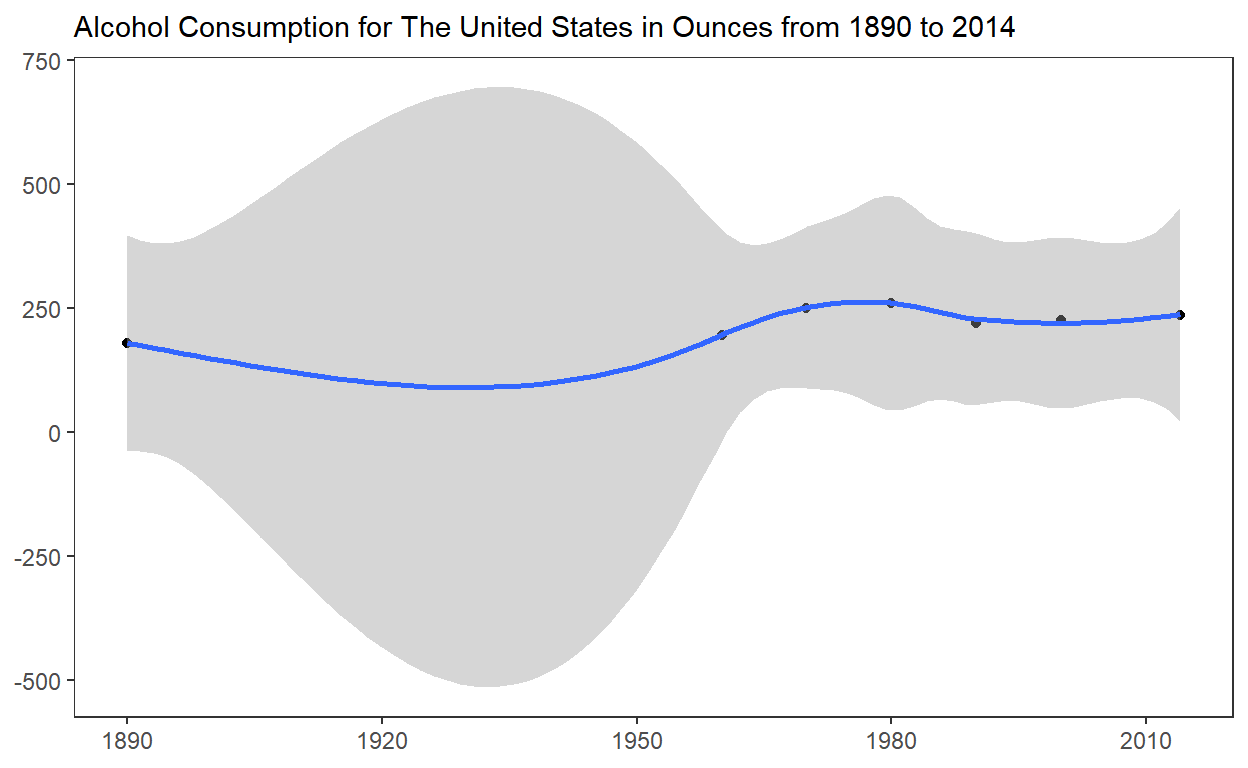# Project Part II

Interactive and Static Plots for Alcohol Consumption in High Income Countries from 1890 to 2014

These are the R packages that I will use to read and plot the data

``````library(tidyverse)
library(echarts4r)
``````

Read the data in from Part I

``````consumption <- read_csv(here::here("consumption.csv"))
``````

## Interactive Graph

• Start with `consumption` THEN

• Use `mutate` to paste `Year` as a character and `round` Ounces to 1 decimal place THEN

• Use `group_by` to group by `Country` THEN

• Use `e_charts` to select `Year` as the object on the x-axis THEN

• Use `e_line` to add a line to the variable `Ounces`

• Set `legend` to FALSE to remove legend from plot
• Use `e-tooltip` to add a tooltip that will display based on axis values THEN

• Use `e_title` to add a title, subtitle and link to subtitle

• Use `e_theme` to change the theme to `dark-fresh-cut`

``````consumption %>%
mutate(Ounces = round(Ounces, 1),
Year = paste(Year, sep = "-")) %>%
group_by(Country) %>%
e_charts(x = Year) %>%
e_line(serie = Ounces, legend = FALSE) %>%
e_tooltip(trigger = "axis") %>%
e_title(text = "Alcohol Consumption from 1890 to 2014 for High Income Countries",
subtext = "In Ounces, Source: Our World in Data",
left = "center") %>%
e_theme("dark-fresh-cut")
``````

This graph displays the alcohol consumption per capita for High Income Countries from 1890 to 2014 in Ounces.

## Static Graph

• Start with `consumption` THEN

• Use `filter` to extract data from `The United States` THEN

• Use `ggplot` to create a plot with data THEN

• Use `geom_point` to add points

• assign `Year` to the x-axis

• assing `Ounces` to the y-axis

• Add a line with `geom_smooth`

• assign `Year` to the x-axis

• assing `Ounces` to the y-axis

• Use `labs` to:

• set subtitle to `Alcohol Consumption for The United States in Ounces from 1890 to 2014`

• set `x` and `y` to NULL so the x and y axis won’t be labeled

• Use `theme_test()` to set the theme

``````consumption %>%
filter(Country == "United States") %>%
ggplot() +
geom_point(aes(x = Year, y = Ounces)) +
geom_smooth(aes(x = Year, y = Ounces)) +
labs(subtitle = "Alcohol Consumption for The United States in Ounces from 1890 to 2014", x = NULL, y = NULL)+
theme_test()
``````Alcohol consumption in The United States of America has been steadily increasing by a few ounces year over year since 1890. Data from 1920 does not exist due to Prohibition.

``````ggsave(filename = "preview.png", path = here::here("_posts", "2022-05-04-project-part-ii"))
``````